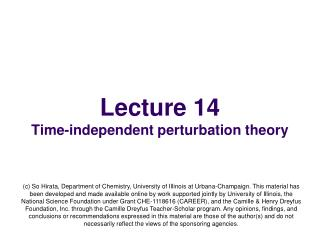DownloadDownload PresentationLecture 14 Time-independent perturbation theory

# Lecture 14 Time-independent perturbation theory

Download Presentation## Lecture 14 Time-independent perturbation theory

- - - - - - - - - - - - - - - - - - - - - - - - - - - E N D - - - - - - - - - - - - - - - - - - - - - - - - - - -
##### Presentation Transcript

1. Lecture 14Time-independent perturbation theory (c) So Hirata, Department of Chemistry, University of Illinois at Urbana-Champaign. This material has been developed and made available online by work supported jointly by University of Illinois, the National Science Foundation under Grant CHE-1118616 (CAREER), and the Camille & Henry Dreyfus Foundation, Inc. through the Camille Dreyfus Teacher-Scholar program. Any opinions, findings, and conclusions or recommendations expressed in this material are those of the author(s) and do not necessarily reflect the views of the sponsoring agencies.

2. Perturbation theory • What is perturbation theory? • To solve complex physical and mathematical problems approximately • We first identify the most closely related problem (zeroth order) with known solutions, • We then adjust the solutions by analyzing the difference. • The main assumption is that the difference is only a small perturbation (disturbance) and we can obtain accurate approximate solutions more easily than solving the problem more exactly.

3. Example of perturbation theory • 10 people went to a bar. Nine (9) of them (Group A) had one drink each and one (1) (Person B) had two drinks. The bill was \$100. • Each paid \$10 (= \$100 / 10 people) (zeroth order solution). • Person B (who had two drinks - perturbation) felt bad and pitched in another \$10 (first order perturbation solution). • There is an overage of \$10 and so \$1 (= \$10 / 10 people) is returned to each (second order perturbation solution). Each in Group A paid \$9 and Person B paid \$19, whereas the exact solution is, each in Group A pays \$100/11 = \$9.0909090909… and Person B pays \$100/11 x 2 = \$18.1818181818…

4. Significance of the theory • The most successful many-body theory. Most of the accurate numerical solutions of the Schrödinger equations (no exact analytical solutions) are based on this theory. • Some of the physical quantities and concepts are based on perturbation theory: dipole moment (1st order), polarizability (2nd order), spin-orbit coupling theory (1st order), one-photon optical transitions (time-dependent 1st order), two-photon optical transitions (time-dependent 2nd order), just to name a few.

5. Zerothorder • Suppose we want to know at least approximately the ground-state solution of the Schrödinger equation • Suppose we have found a similar problem with a known solution The ground state Known solution

6. Partitioning of Hamiltonian • First we view H as the sum of H(0) and a perturbation λ is equal to 1, and has no effect except it helps us classify terms 0th-order part The greater the number in parenthesis, the smaller the term. This term is a small perturbation as compared to (0) term Perturbation So far no approximation yet

7. Series expansions • If we “turn on” H(1)in the Hamiltonian, the wave function and energy change – the size of change that is linear, quadratic, cubic, etc. to the size of H(1) (cf. Taylor expansion) If we carry through this infinite summation, we get the exact solution of HΨ0=E0Ψ0. So far, no approximation has been made.

8. Sizes of terms • The powers in λindicate the relative sizes of terms. • For example, if the perturbation H(1) is 1% of H(0) in size, Ψ(1) is also roughly 1% of Ψ(0) and then Ψ(2) is 1% x 1% = 0.0001 of Ψ(0). • We equate terms of roughly equal sizes. So, for example, the terms having λ1and λ2are very different in size and should not be compared.

9. The Schrödinger equation • Substitute the Hamiltonian, wave function, energy into the Schrödinger equation that we intend to solve (not the zeroth-order one we already know the solution of).

10. Order-by-order equations • Equating the terms containing the same powers of λ, This is nothing but the zeroth-order equation we started with.

11. First order • Repeating this process, we obtain an infinite series of corrections the sum of which converge at the exact solution. Truncating the series constitutes an approximation. • For example, if we stop at a finite order and solve the following first-order equation, then we obtain E(0) + E(1)as an approximation to true E.

12. First order • Now we expand the unknown wave function Ψ0(1) as a linear combination of known wave functions {Ψn(0)}. This is always possible because of the completeness of eigenfunctions of an Hermitian operator. {Ψn(0)} [ground- and all excited-state wave functions of H(0)] forms a complete set.

13. First order • Substituting Multiply or (k > 0) from the left and integrate over space

14. First order • From the first equation: 1 cancel exactly cancel exactly c0 contribution cancels exactly and it is simply repeating the λ0 equation

15. First order • From the second equation 0

16. Second order Multiply from the left and integrate over space cancel 1

17. First order • This looks like an expectation value of the perturbation operator.

18. Second order Excited De-excited 1st-order WF Excited - deexcited

19. Summary • Perturbation theory works as follows: • Find a suitable zeroth-order Hamiltonian (close to the true Hamiltonian) whose eigenfunctionsand eigenvalues are known. • Partition the Hamiltonian into the zeroth-order part and perturbation. • Expand the energy and wave function into series of smaller and smaller corrections to zeroth-order quantities. • Equate the terms according to the sizes. • Use completeness, normalization,and orthogonalityof zeroth-order eigenfunctions to obtain general expressions for energies and wave functions.